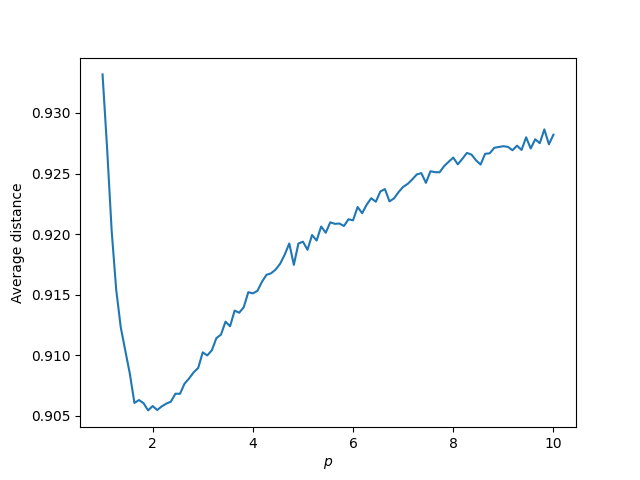# Average lp distance in a disk

This post will define ℓp distance and look at the average ℓp distance between points in the ℓp unit disk. We will compute this distance by simulation.

## ℓp distance

The ℓp distance between two points (x1, y1) and (x2, y2) isThe ℓp unit disk is the points whose ℓp distance from the origin is less than or equal to 1.

When p = 2, ℓp distance is Euclidean distance and the ℓp unit disk is the ordinary unit disk.

When p = 1 the distance between two points is the sum of the absolute differences in their coordinates and the unit disk is a diamond.

For p = ∞ the distance if defined by the limit of the ℓp distance, which works out to be the maximum absolute difference in any two coordinates. The unit disk becomes a square.

## Average distance

Suppose we pick two points uniformly from an ℓp disk and ask how far apart they are in ℓp distance. The expected value is studied in . The authors give exact values for a few special cases.

When p = 1 or p = ∞ the average distance is 14/15. When p = 2 the average distance is 128/45π = 0.9054.

This suggests that the average distance decreases at first then at some point it starts increasing again. Where’s the minimum? Is there only one local minimum?

A pair of exponents (p, q) is said to be conjugate

1/p + 1/q = 1

which is the case for (1, ∞). From this scant bit of evidence I’d conjecture that the average distance is the same for conjugate exponents. If so, there’s a sort of symmetry around p = 2 and so perhaps the minimum average distance occurs at p = 2.

## Simulation

The following Python code estimates the average distances described above using simulation. The code is meant to be clear rather than efficient. A much more efficient approach would be to use numerical integration rather than simulation. But the simulation approach maps directly onto the problem at hand and can be written quickly. Simulation is efficient for me; integration would be efficient for the computer.

The code uses acceptance-rejection sampling to generate points in the ℓp disk: it generates points from a square around the disk and throws away points until it gets a point inside the disk.

    import numpy as np

def in_disk(pt, p):
return abs(pt)**p + abs(pt)**p <= 1

def random_point_in_square():
# random point in [-1, 1] x [-1, 1]
return 2*np.random.random(2) - np.array([1,1])

def random_point_in_disk(p):
pt = random_point_in_square()
while not in_disk(pt, p):
pt = random_point_in_square()
return pt

def distance(u, v, p):
w = u - v
return (abs(w)**p + abs(w)**p)**(1/p)

def avg_distance(p, N):
s = 0
for _ in range(N):
u = random_point_in_disk(p)
v = random_point_in_disk(p)
s += distance(u, v, p)
return s/N


When I ran this code with N = 1,000,000 and p = 1 and 2, I got 0.932 and 0.906 respectively. With this value of N we would expect about three digit accuracy, which is what we got.

## Varying p

The following plot was made by setting N = 1,000,000 and varying p from 1 to 10.The code above is slow. I wrote the rest of this post while waiting for the plot to finish. In particular, I made my conjecture above before seeing the plot. It seems that the minimum may indeed be at p = 2, and that the curve is monotonic on either side. (The jaggedness of the plot is presumably an artifact of simulation.)

To test whether conjugate exponents have the same average distance, I increased N to 10,000,000 and printed out the values for p = 1.5 and p = 3. I got average distances 0.9100059 and 0.9100589. The difference between the two values is well within what we’d expect given our value of N.

So the simulation results are consistent with my conjecture. I may try to prove the conjecture analytically or speed up the numerical results with integration.

 C. K. Wong and Kai-Ching Chu. Distances in lp Disks. SIAM Review, Vol. 19, No. 2 (Apr., 1977), pp. 320-324.

## 3 thoughts on “Average lp distance in a disk”

1. Matthew Doar

I enjoyed this, thanks.

“The code is not meant to be clear” – perhaps you meant “The code is meant to be clear”?